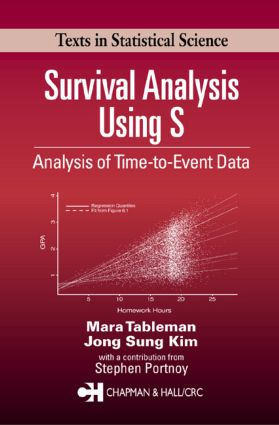Survival Analysis Using S

Analysis of Time-to-Event Data, 1st Edition

Chapman and Hall/CRC

280 pages | 69 B/W Illus.

Purchasing Options:\$ = USD
Hardback: 9781584884088
pub: 2003-07-28
SAVE ~\$26.00
\$130.00
\$104.00
x

FREE Standard Shipping!

Description

Survival Analysis Using S: Analysis of Time-to-Event Data is designed as a text for a one-semester or one-quarter course in survival analysis for upper-level or graduate students in statistics, biostatistics, and epidemiology. Prerequisites are a standard pre-calculus first course in probability and statistics, and a course in applied linear regression models. No prior knowledge of S or R is assumed. A wide choice of exercises is included, some intended for more advanced students with a first course in mathematical statistics.

The authors emphasize parametric log-linear models, while also detailing nonparametric procedures along with model building and data diagnostics. Medical and public health researchers will find the discussion of cut point analysis with bootstrap validation, competing risks and the cumulative incidence estimator, and the analysis of left-truncated and right-censored data invaluable. The bootstrap procedure checks robustness of cut point analysis and determines cut point(s).

In a chapter written by Stephen Portnoy, censored regression quantiles - a new nonparametric regression methodology (2003) - is developed to identify important forms of population heterogeneity and to detect departures from traditional Cox models. By generalizing the Kaplan-Meier estimator to regression models for conditional quantiles, this methods provides a valuable complement to traditional Cox proportional hazards approaches.

Reviews

"All in all the book succeeds nicely in getting the reader through the basic methods of survival analysis (Kaplan-Meier, log-rank, Weibull and Cox regression) and how to implement them in S."

-Journal of Statistical Software, Vol. 11, July 2004

"There are many books on survival analysis, so an obvious question is what makes this one any different …? The main answers are the well-integrated S code that is used throughout the book and a chapter on censored regression quantiles … . [T]he topics that are covered … provide the reader with a good grasp of the principles of analysing survival data and the writing style is clear and easy to follow. I recommend this book for anyone who wants a good introduction to practical survival analysis using S."

-Journal of the Royal Statistics Society, Issue 167(4)

"This book introduces the field of survival analysis in a concise, coherent manner that capture the spirit of the methods without getting too embroiled in theoretical technicalities…this well-written book would be an excellent choice for a textbook for a course in survival analysis."

-Zentralblatt MATH 104

"This well-written book would be an excellent choice for a textbook for a course in survival analysis. All of the usual topics for a course in survival analysis are covered, including a careful discussion of parametric models. The explanations are clear and concise. The book not only teaches about the statistical methods for survival analysis, but also provides detailed instruction on how to do the computations with S-PLUS or R at a level where students will become proficient with the S language. The book contains an excellent collection of exercises. These exercises have been usefully partitioned into applications and questions that ask students to use their knowledge of probability and mathematical statistics."

-William Q Meeker, Distinguished Professor in the Department of Statistics, Iowa State University

INTRODUCTION

Motivation - Two Examples

Basic definitions

Censoring and Truncation Models

Course Objectives

Data entry and Import/Export of Data Files

Exercises

NONPARAMETRIC METHODS

Kaplan-Meier Estimator of Survival

Comparison of Survivor Curves: Two-Sample Problem

Exercises

PARAMETRIC METHODS

Frequently Used (Continuous) Models

Maximum Likelihood Estimation (MLE)

Confidence Intervals and Tests

One-Sample Problem

Two-Sample Problem

A Bivariate Version of the Delta Method

The Delta Method for a Bivariate Vector Field

General Version of the Likelihood Ratio Test

Exercises

REGRESSION MODELS

Exponential Regression Model

Weibull Regression Model

Cox Proportional Hazards (PH) Model

Accelerated Failure Time Model

Summary

AIC procedure for Variable Selection

Exercises

THE COX PROPORTIONAL HAZARDS MODEL

AIC Procedure for Variable Selection

Stratified Cox PH Regression

Exercises

Review of First Five Chapters: Self-Evaluation

MODEL CHECKING: DATA DIAGNOSTICS

Basic graphical Methods

Weibull Regression Model

Cox proportional Hazards Model

Exercises

Extended Cox Model

Competing Risks: Cumulative Incidence Estimator

Analysis of Left-Truncated and Right-Censored Data

Exercises

CENSORED REGRESSION QUANTILES, by Stephen Portnoy

Introduction

What are Regression Quantiles?

Computation of Censored Regression Quantiles

Examples of Censored Regression Quantile

Exercises

REFERENCES

INDEX